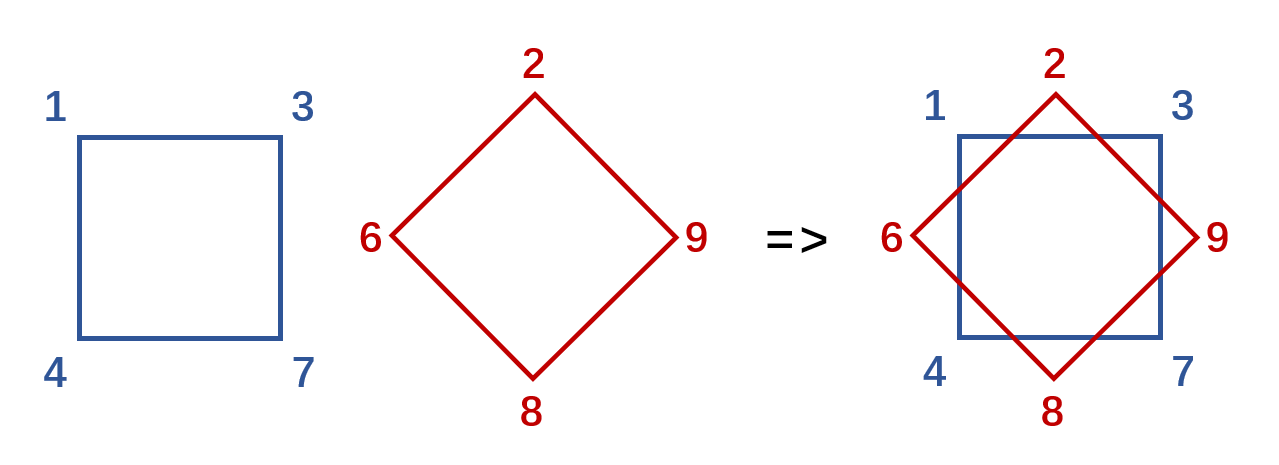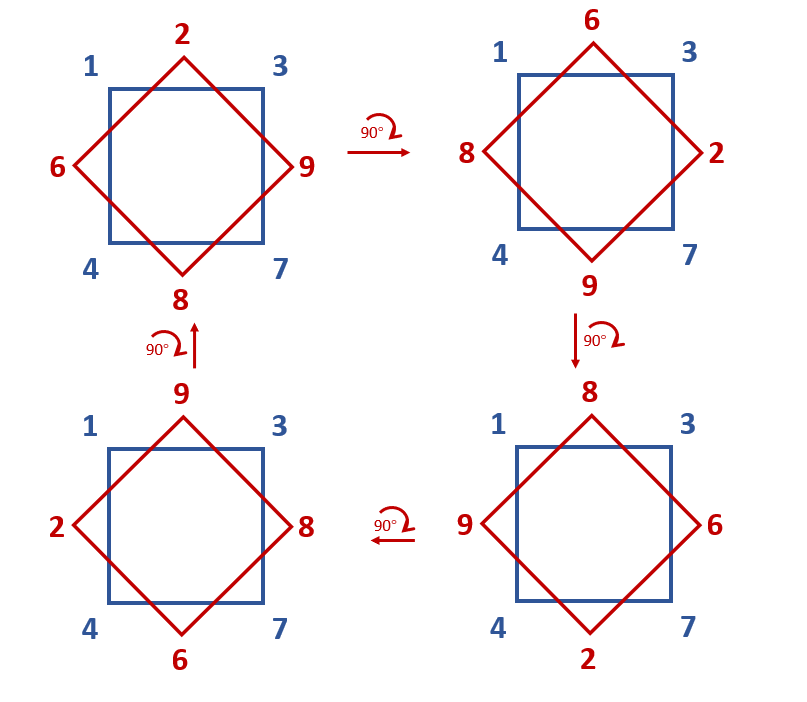# 前置知识·

## 置换群·

$\sigma = \begin{pmatrix} 1 & 2 & 3 \\ 2 & 3 & 1 \end{pmatrix}$

$\sigma(1) = 2 \\ \sigma(2) = 3 \\ \sigma(3) = 1$

## 群操作·

$(\sigma \circ \tau)(x) = \sigma(\tau(x))$

## 回圈表述·

$\sigma(a_0) = a_1 \\ \sigma(a_1) = a_2 \\ \vdots \\ \sigma(a_{k-1}) = a_0$

PS：有的文章也会从下标为$1$$a_1$开始，不过我习惯$0$开始，而且一会也更好说明

$\sigma = \begin{pmatrix} 1 & 2 & 3 & 4 & 5 & 6 & 7 \\ 2 & 4 & 1 & 3 & 6 & 5 & 7 \end{pmatrix}$

# 置换群开方·

## 平方操作·

$\sigma = \begin{pmatrix} 1 & 2 & 3 & 4 & 5 & 6 & 7 \\ 2 & 4 & 1 & 3 & 6 & 7 & 5 \end{pmatrix} = (1,2,4,3)(5,6,7)$

$\begin{array}{c|ccccccc|c} x & 1 & 2 & 3 & 4 & 5 & 6 & 7 & () \\ \sigma(x) & 2 & 4 & 1 & 3 & 6 & 7 & 5 & (1,2,4,3)(5,6,7) \\ \sigma^2(x) & 4 & 3 & 2 & 1 & 7 & 5 & 6 & (1,4)(2,3)(5,7,6) \end{array}$

$x=1$做栗子，本来$\sigma$中是$1 \to 2$$2 \to 4$，现在$\sigma^2$是连着做两次$\sigma$，即$1 \to 2 \to 4$，省略中间过程的$2$，即$1 \to 4$，其他情况类似，可以看上表的最后一行

$\sigma_i^2(a_0) = a_2 \\ \sigma_i^2(a_1) = a_3 \\ \vdots \\ \sigma_i^2(a_j) = a_{j + 2 \pmod k}$

• 如果$k$是偶数，由于$\text{GCD}(2, k) = 2$，所以$\sigma_i^2$会被拆分为$\sigma_i^2 = (a_0, a_2, \cdots, a_{k-2})(a_1, a_3, \cdots, a_{k-1})$两个圈，这两个圈的长度相同且都为$\frac{k}{2}$
• 如果$k$是奇数，由于$\text{GCD}(2, k) = 1$，所以$\sigma_i^2$还是一个长度为$k$的圈，只是里面元素的顺序会改变，变为$\sigma_i^2 = (a_0, a_2, \cdots, a_{k-3}, a_{k-1}, a_1, a_3, \cdots, a_{k-4}, a_{k-2})$

## 开方操作·

### 奇数圈·

$\tau_i = (r_0, r_1, \cdots, r_{k-1}) \\ \sigma_j = (a_0, a_1, \cdots, a_{k-1})$

\begin{aligned} \tau_i &= \sigma_j^2 \\ &= (a_0, a_2, \cdots, a_{k-3}, a_{k-1}, a_1, a_3, \cdots, a_{k-4}, a_{k-2}) \\ &= (r_0, r_1, \cdots, r_{k-1}) \end{aligned}

$\begin{array}{cccccccccc} a_0 & a_2 & \cdots & a_{k-3} & a_{k-1} & a_1 & a_3 & \cdots & a_{k-4} & a_{k-2} \\ r_0 & r_1 & \cdots & r_{\frac{k-1}{2}-1} & r_{\frac{k-1}{2}} & r_{\frac{k-1}{2} + 1} & r_{\frac{k-1}{2} + 2} & \cdots & r_{k-2} & r_{k-1} \end{array}$

$\begin{array}{cccccc} a_0 & a_2 & \cdots & \cdots & a_{k-3} & a_{k-1} \\ r_0 & r_1 & \cdots & \cdots & r_{\frac{k-1}{2}-1} & r_{\frac{k-1}{2}} \\ \hline a_1 & a_3 & \cdots & a_{k-4} & a_{k-2} \\ r_{\frac{k-1}{2} + 1} & r_{\frac{k-1}{2} + 2} & \cdots & r_{k-2} & r_{k-1} \end{array}$

$a$行的顺序排一下即得$\sigma_j = (r_0, r_{\frac{k-1}{2} + 1}, r_1, r_{\frac{k-1}{2} + 2}, \cdots, r_{\frac{k-1}{2} - 1}, r_{k-1}, r_{\frac{k-1}{2}})$，这在数学上表达会有点麻烦，但在代码中就是上面取一个下面取一个这样就好了，比如

### 偶数圈·

$\tau_{i_0} = (r_{0, 0}, r_{0, 1}, \cdots, r_{0, \frac{k}{2}-1}) \\ \tau_{i_1} = (r_{1, 0}, r_{1, 1}, \cdots, r_{1, \frac{k}{2}-1}) \\ \sigma_j = (a_0, a_1, \cdots, a_{k-1})$

\begin{aligned} \tau_{i_0} \tau_{i_1} &= \sigma_j^2 = (a_0, a_2, \cdots, a_{k-2})(a_1, a_3, \cdots, a_{k-1}) \\ \tau_{i_0} &= (a_0, a_2, \cdots, a_{k-2}) \\ \tau_{i_1} &= (a_1, a_3, \cdots, a_{k-1}) \end{aligned}

$\tau_{i_0} = (r_{0, 0}, r_{0, 1}, \cdots, r_{0, \frac{k}{2}-1}) = (a_0, a_2, \cdots, a_{k-2}) \\ \tau_{i_1} = (r_{1, 0}, r_{1, 1}, \cdots, r_{1, \frac{k}{2}-1}) = (a_1, a_3, \cdots, a_{k-1}) \\$

$(b_0, b_1, b_2) = (b_1, b_2, b_0) = (b_2, b_0, b_1)$

$\tau_{i_0} = (1,3,7,4) \\ \tau_{i_1} = (2,9,8,6)$

$(a_0, a_2, a_4, a_6) = (1,3,7,4) \\ (a_1, a_3, a_5, a_7) = (2,9,8,6)$

$(a_0, a_2, a_4, a_6) = (1,3,7,4) \\ (a_1, a_3, a_5, a_7) = (9,8,6,2)$$\tau_{i_0} \tau_{i_1} = \sigma_j^2 = (r_{0, 0}, r_{0, 1}, \cdots, r_{0, \frac{k}{2}-1}) (r_{1, 0}, r_{1, 1}, \cdots, r_{1, \frac{k}{2}-1})$

\begin{aligned} \tau_{i_0} &= (r_{0, 0}, r_{0, 1}, \cdots, r_{0, \frac{k}{2}-1}) \\ &= (a_0, a_2, \cdots, a_{k-2}) \\ \tau_{i_1} &= (r_{1, 0}, r_{1, 1}, \cdots, r_{1, \frac{k}{2}-1}) \\ &= (a_{1+2l \pmod {\frac{k}{2}}}, a_{3+2l \pmod {\frac{k}{2}}}, \cdots, a_{k-1+2l \pmod {\frac{k}{2}}}) \\ \end{aligned}

$(a_1, a_3, \cdots, a_{k-1}) = (r_{1, 0+l \pmod {\frac{k}{2}}}, r_{1, 1+l \pmod {\frac{k}{2}}}, \cdots, r_{1, \frac{k}{2}-1+l \pmod {\frac{k}{2}}})$

$\begin{array}{cccc} a_0 & a_2 & \cdots & a_{k-2} \\ r_{0, 0} & r_{0, 1} & \cdots & r_{0, \frac{k}{2}-1} \\ \hline a_1 & a_3 & \cdots & a_{k-1} \\ r_{1, 0+l \pmod {\frac{k}{2}}} & r_{1, 1+l \pmod {\frac{k}{2}}} & \cdots & r_{1, \frac{k}{2}-1+l \pmod {\frac{k}{2}}} \end{array}$

# BRICS+的sqrt·

• 长度$75$的明显是某个长度$75$的回圈平方所得
• 那两个长度$82$的也明显是某个长度$164$的回圈平方所得
• $3$个长度为$3$的就有点麻烦，因为可能三个都是奇数圈平方，也有可能只有一个是奇数圈平方，另外两个是偶数圈平方
• $8$个长度$1$的就更麻烦了

PS：这只是针对输入的代码，通解的代码好像有点麻烦，就先留坑了

PPS：set()会有个坑，就是每次输出的顺序都是不一样的，导致复现的时候时间不准，后面加了sorted测出顺序爆破24:42，逆序19:43，完整爆破40min+，可以考虑多线程了（阴间题

# 参考·

• Judson T W. Abstract algebra: theory and applications[M]. 2020.
• https://math.stackexchange.com/questions/266569/how-to-find-the-square-root-of-a-permutation